# Region and Edge Based Segmentation

## Introduction

Image Segmentation is the process of dividing a digital image into smaller groups so that processing and analyzing the larger images becomes easier and simpler. Region and Edge-based segmentation are different types of Image Segmentation.

Before diving into Region and Edge based Segmentation, let us have a brief overview of how segmentation is done.

## Image Segmentation

In simpler terms, segmentation is the process of assigning specific labels to pixels in an image. A group of pixels with the same label become a segment of the larger image.

For example, below are two images with their segmentation.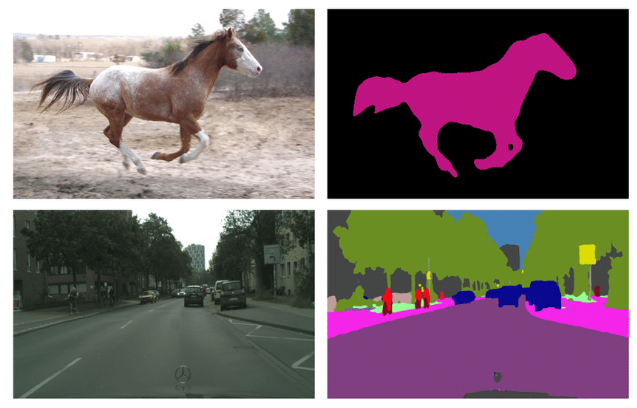In the first image, the picture of the horse is a single segment separated from its surrounding.

In the second image, the road on the left side is divided digitally into different segments on the right side and is shown with different colors.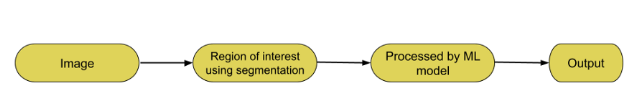Thus, this process reduces the complexity of computation and processing, especially for image processing and AI algorithms, since now we can work on segment levels rather than processing the whole image at once which can be highly intensive on computation and a strain on resources.

Now, that we have learned some basics of segmentation, let us move on to Region and Edge- based segmentation.

## Region Based Segmentation

This process involves dividing the image into smaller segments that have a certain set of rules. This technique employs an algorithm that divides the image into several components with common pixel characteristics. The process looks out for chunks of segments within the image. Small segments can include similar pixes from neighboring pixels and subsequently grow in size. The algorithm can pick up the gray level from surrounding pixels.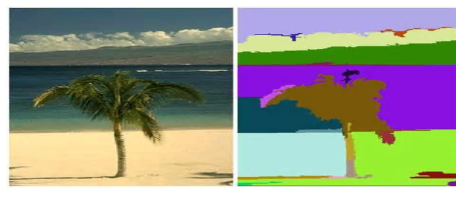They are two types

• Region growing − This method recursively grows segments by including neighboring pixels with similar characteristics. It uses the difference in gray levels for gray regions and the difference in textures for textured images.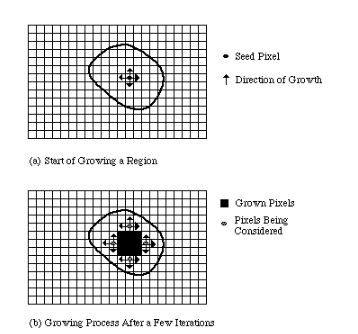• Region splitting − In this method, the whole image is considered a single region. Now to divide the region into segments it checks for pixels included in the initial region if they follow the predefined set of criteria. If they follow similar rules they are taken into one segment.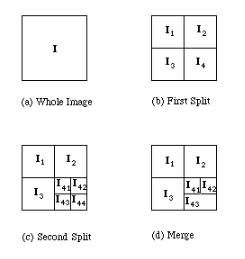## Edge Based Segmentation

In Edge Based segmentation, the boundaries or edges of the images are significantly different from each other and also from the background of the image. This fact is used to do edge detection on images with different levels of intensities and discontinuity on edges. Edges have quite a good amount of information about the image. Two common tasks in this method are edge detection and edge linking. In edge detection, the boundary is identified. Edge linking as the name suggests involves linking one edge with another. A common technique used to detect contours is thresholding.Now, let us look into a code implementation of edge and region segmentation.

## Code Implementation – Edge-based

### Example

import numpy as np
import matplotlib.pyplot as plt
from skimage import data
from skimage.feature import canny
from scipy import ndimage as ndi

coins = data.coins()
h = np.histogram(coins, bins=np.arange(0, 256))
figure, (ax_1) = plt.subplots()
ax_1.imshow(coins, cmap=plt.cm.gray)

edges = canny(coins/255.)
figure, axis = plt.subplots(figsize=(6, 4))
axis.imshow(edges, cmap=plt.cm.gray)
axis.axis('off')
axis.set_title('Detect canny')

fc = ndi.binary_fill_holes(edges)
figure, axis = plt.subplots(figsize=(6, 4))
axis.imshow(fc, cmap=plt.cm.gray)
axis.axis('off')
axis.set_title('hole fill')


### Output

Text(0.5, 1.0, 'hole fill')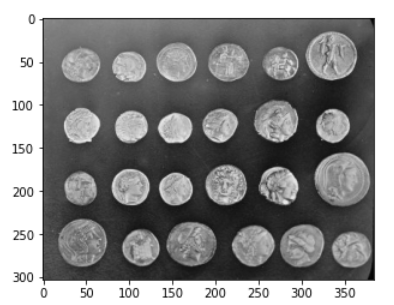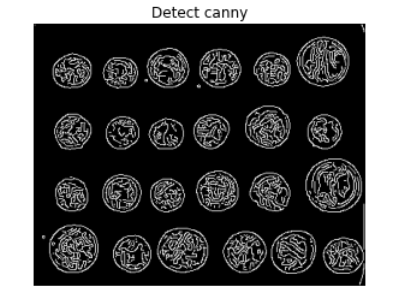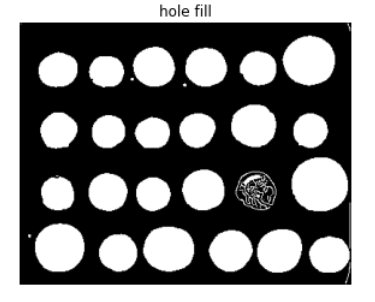## Code Implementation – Region-based

### Example

from skimage.filters import sobel
import numpy as np
import matplotlib.pyplot as plt
from skimage import data
from skimage.feature import canny
from scipy import ndimage as ndi

coins = data.coins()
elmap = sobel(coins)
figure, axis = plt.subplots(figsize=(6, 4))
axis.imshow(elmap, cmap=plt.cm.gray)
axis.axis('off')
axis.set_title('map elevation')


### Output

Text(0.5, 1.0, 'map elevation')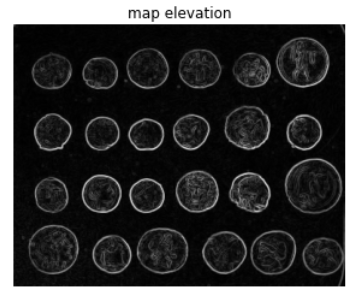## Conclusion

Region and Edge-based segmentation are useful in modern algorithms which rely on Image and video-based operations. These are simple techniques but highly powerful.